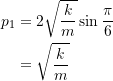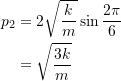### Advanced Dynamics and Vibrations: Numerical methods for vibrating systems

[Jason’s part goes here]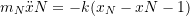where we can have a different mass as the equation is different. For SHM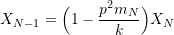If we choose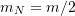then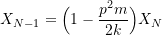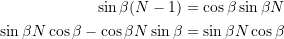Therefore: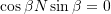Note: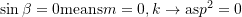If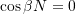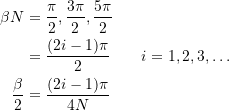Now using the result above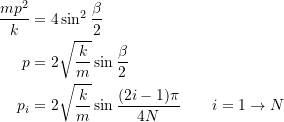Consider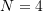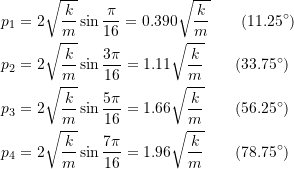Note:We can apply this idea to various dynamical systems which are more complicated than the simple spring/mass. Consider an approximation to longitudinal vibration of a bar

Consider a beam of total mass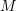and cross section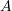modulus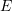which we approximate as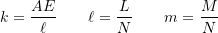if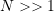, go to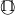if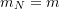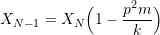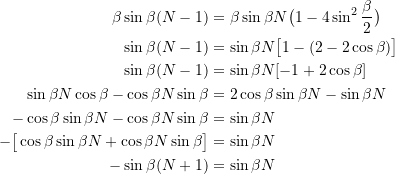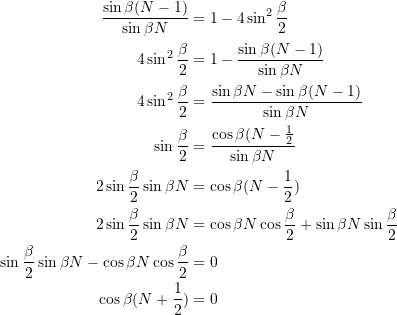Therefore: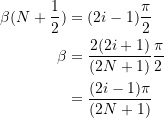Therefore: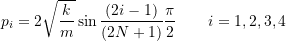N = 4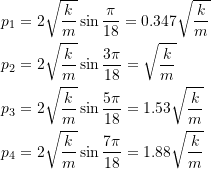To calculate the mode shapes one can start at the free end e.g.: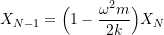Assume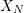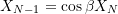Now we use the recursion relationship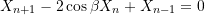Therefore: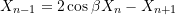For the 4 storey building for the lowest natural frequency is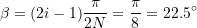Therefore: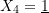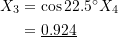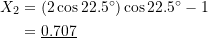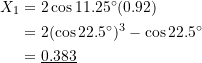Check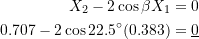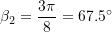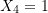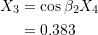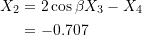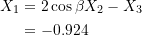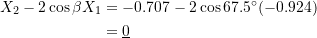Therefore: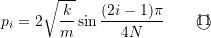if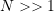(is low) then: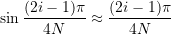for the lower frequencies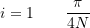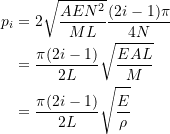where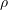is density

For other boundary conditions, the expression for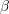is determined then the natural frequencies are known. For fixed ends.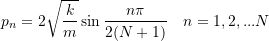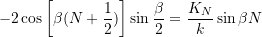Consider the fixed boundaries: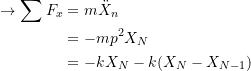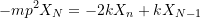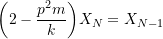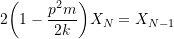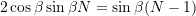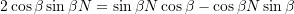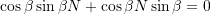Therefore: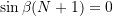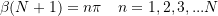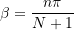Therefore: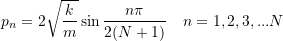##### Example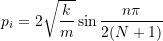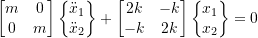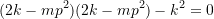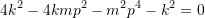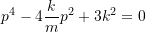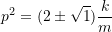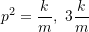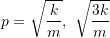Therefore: